Beams Index

Singularity Functions

Introduction

When calculating the shear Force and the bending moment diagrams for more complex loading across discontinuities such as concentrated loads and moments.  Simple methods are not enough.  For the more complicated cases the use of singularity functions provide a convenient method.

A singularity function is expressed as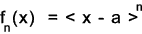Where
n = any integer (positive or negative) including zero
a = distance on x axis along the beam,from the selected origin, identifying the location of the discontinuity.

Rules in applying singularity functions
 If n >0 and the expression inside the angular brackets is positive then fn(x) = (x-a)n the expression is a normal algbraic formula If n > 0 and the expression inside the angular brackets is negative then fn(x) = 0 If n < 0 then fn = 1 for x = a and fn(x) = 0 otherwise If n = 0 then fn = 1 for x >= a and fn(x) = 0 otherwise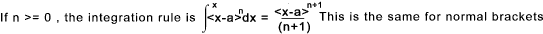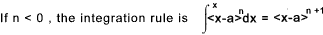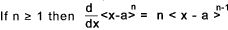Unit Singularity Function Singularity Function as used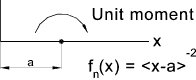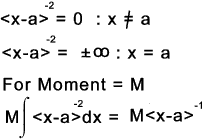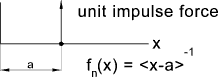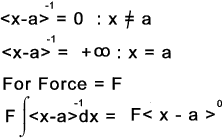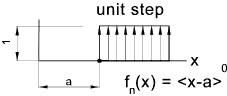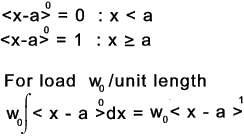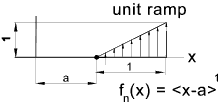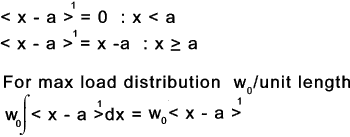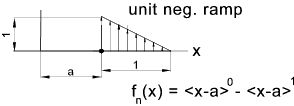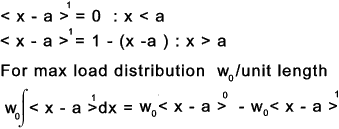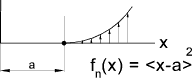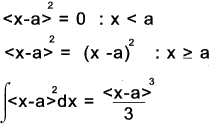Example of using singularity functions for a simply supported beam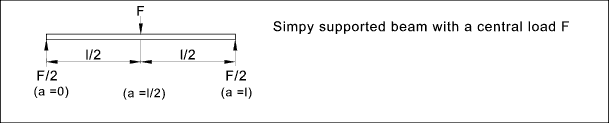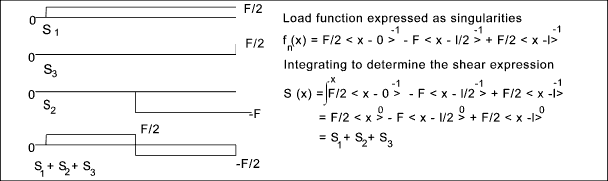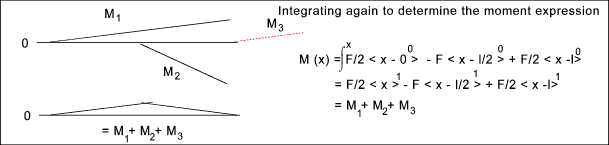A more complex example of using singularity functions

The requirement is to obtain the Shear load, moment, slope and deflection anywhere along the beam as shown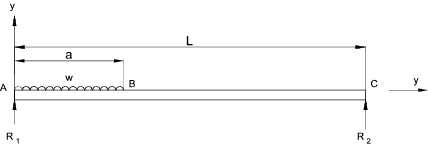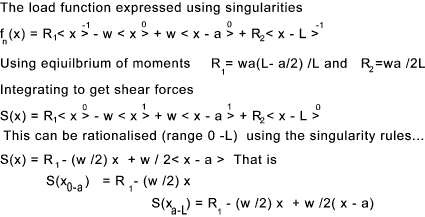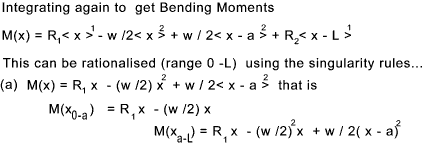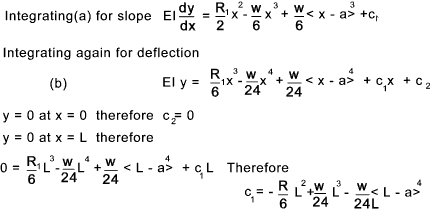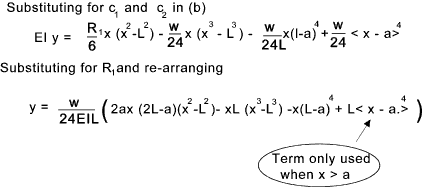The equations above can be used to determine the shear load, moment, slope and deflection for the beam from x = 0 to x = L

 Sites Providing Relevant Information Beam deflection usingDiscontinuity Functions...pdf download - Very informative paper Deflection of Beams...Berkely U. Useful download on beam deflection including use of singularity functions Singularity� functions-Notes from office hours... Concise information with comments
Beams Index